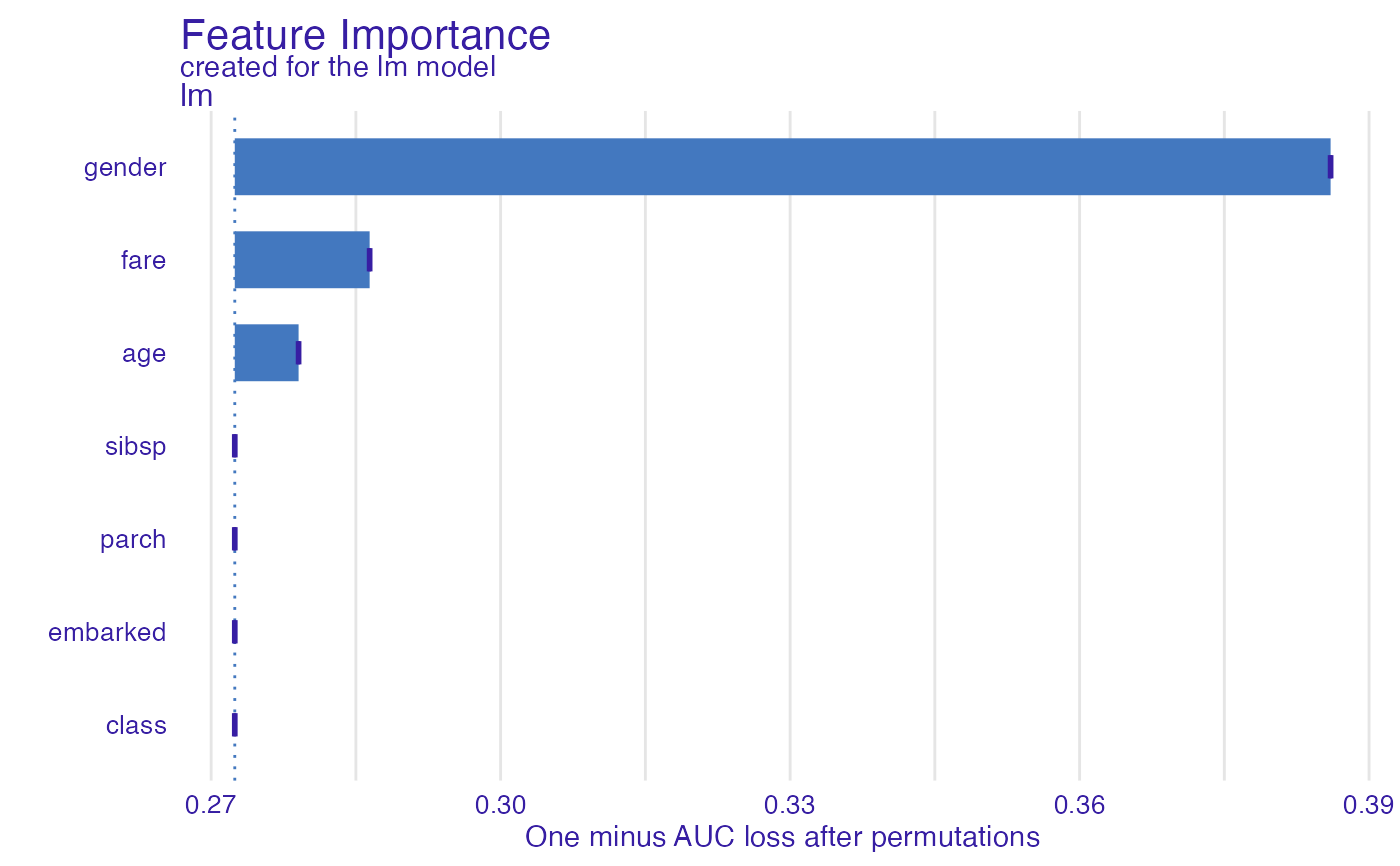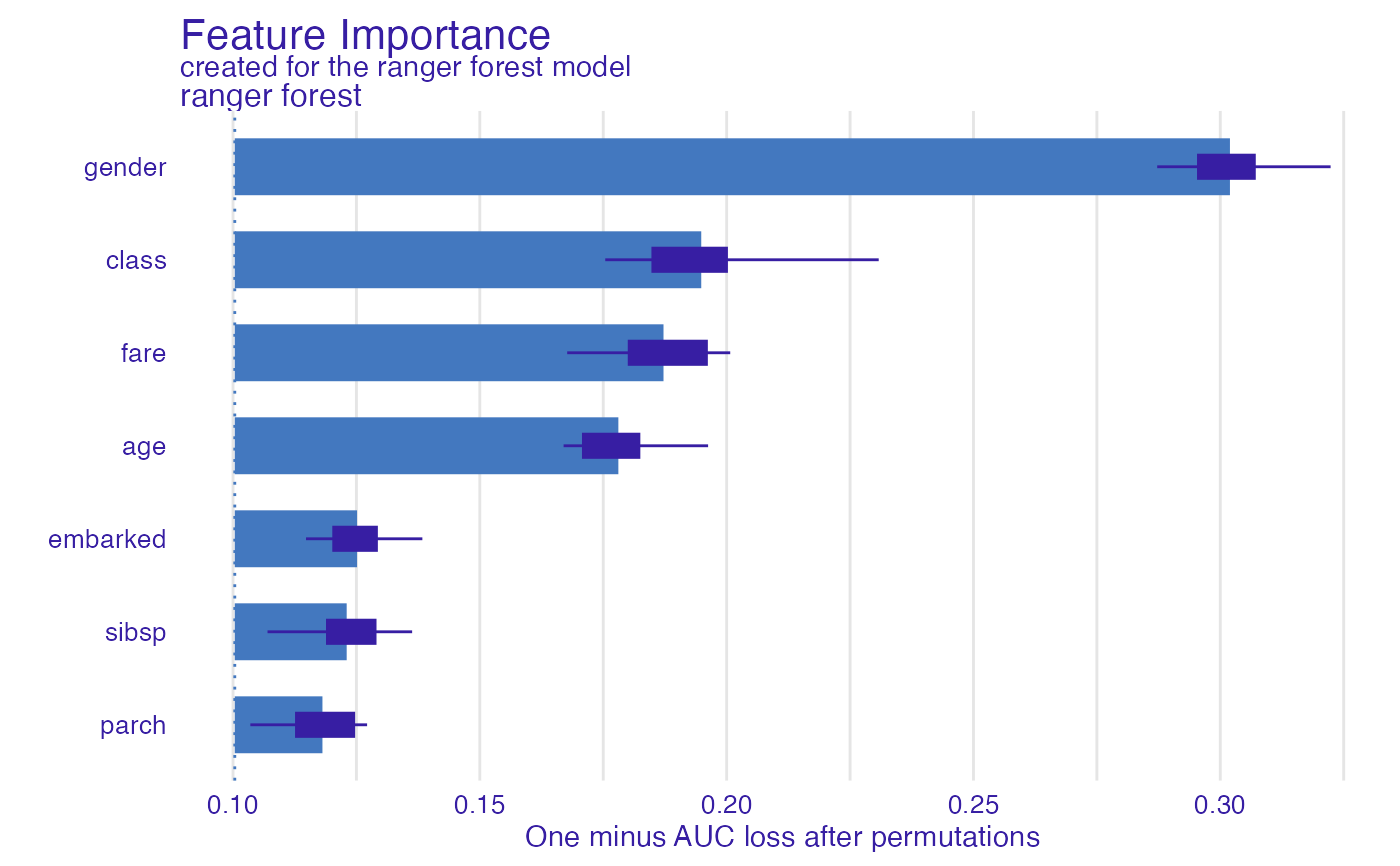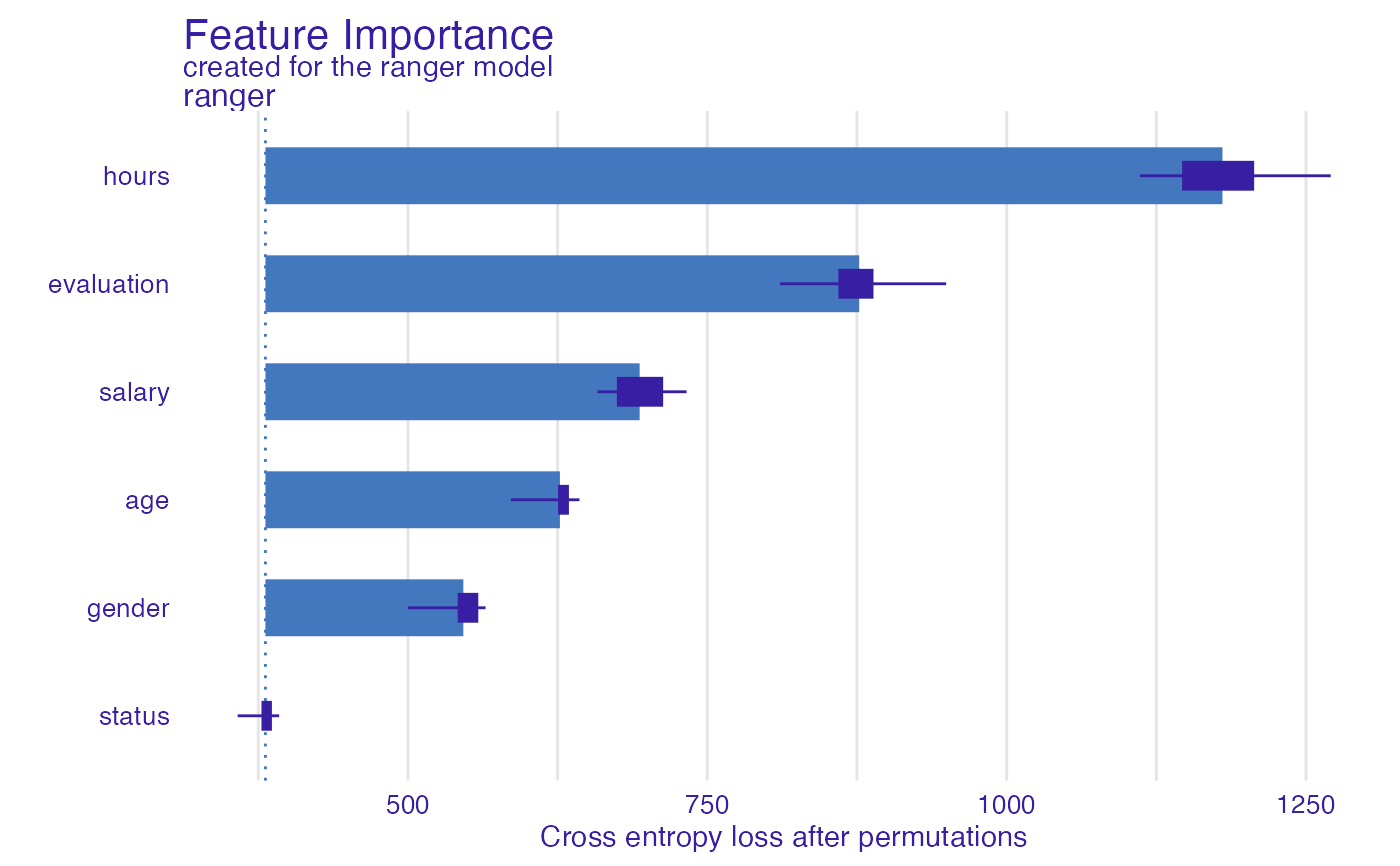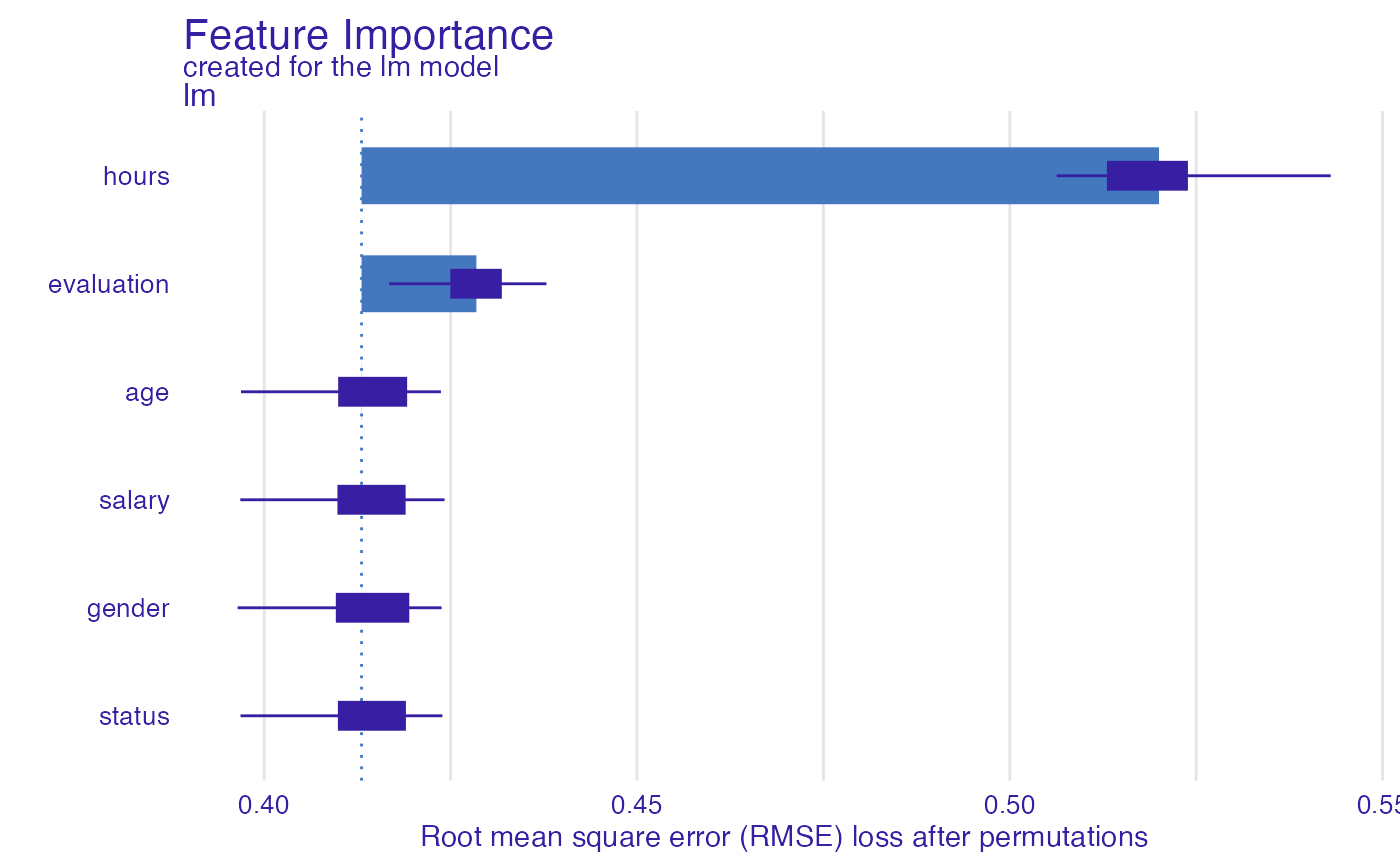This function plots variable importance calculated as changes in the loss function after variable drops. It uses output from feature_importance function that corresponds to permutation based measure of variable importance. Variables are sorted in the same order in all panels. The order depends on the average drop out loss. In different panels variable contributions may not look like sorted if variable importance is different in different in different models.

# S3 method for feature_importance_explainer
plot(
x,
...,
max_vars = NULL,
show_boxplots = TRUE,
bar_width = 10,
desc_sorting = TRUE,
title = "Feature Importance",
subtitle = NULL
)

## Arguments

x a feature importance explainer produced with the feature_importance() function other explainers that shall be plotted together maximum number of variables that shall be presented for for each model. By default NULL what means all variables logical if TRUE (default) boxplot will be plotted to show permutation data. width of bars. By default 10 logical. Should the bars be sorted descending? By default TRUE the plot's title, by default 'Feature Importance' the plot's subtitle. By default - NULL, which means the subtitle will be 'created for the XXX model', where XXX is the label of explainer(s)

## Value

a ggplot2 object

## Details

Find more details in the Feature Importance Chapter.

Explanatory Model Analysis. Explore, Explain, and Examine Predictive Models. https://ema.drwhy.ai/

## Examples

library("DALEX")
library("ingredients")

model_titanic_glm <- glm(survived ~ gender + age + fare,
data = titanic_imputed, family = "binomial")

explain_titanic_glm <- explain(model_titanic_glm,
data = titanic_imputed[,-8],
y = titanic_imputed[,8])
#> Preparation of a new explainer is initiated
#>   -> model label       :  lm  (  default  )
#>   -> data              :  2207  rows  7  cols
#>   -> target variable   :  2207  values
#>   -> predict function  :  yhat.glm  will be used (  default  )
#>   -> predicted values  :  No value for predict function target column. (  default  )
#>   -> model_info        :  package stats , ver. 4.1.1 , task classification (  default  )
#>   -> predicted values  :  numerical, min =  0.1490412 , mean =  0.3221568 , max =  0.9878987
#>   -> residual function :  difference between y and yhat (  default  )
#>   -> residuals         :  numerical, min =  -0.8898433 , mean =  4.198546e-13 , max =  0.8448637
#>   A new explainer has been created!
fi_rf <- feature_importance(explain_titanic_glm, B = 1)
plot(fi_rf)# \donttest{
library("ranger")
model_titanic_rf <- ranger(survived ~., data = titanic_imputed, probability = TRUE)

explain_titanic_rf <- explain(model_titanic_rf,
data = titanic_imputed[,-8],
y = titanic_imputed[,8],
label = "ranger forest",
verbose = FALSE)

fi_rf <- feature_importance(explain_titanic_rf)
plot(fi_rf)HR_rf_model <- ranger(status~., data = HR, probability = TRUE)

explainer_rf  <- explain(HR_rf_model, data = HR, y = HR$status, verbose = FALSE, precalculate = FALSE) fi_rf <- feature_importance(explainer_rf, type = "raw", max_vars = 3, loss_function = DALEX::loss_cross_entropy) head(fi_rf) #> variable mean_dropout_loss label #> 1 _full_model_ 380.9728 ranger #> 2 status 380.9728 ranger #> 3 gender 546.2558 ranger #> 4 age 626.9204 ranger #> 5 salary 693.4965 ranger #> 6 evaluation 876.8476 rangerplot(fi_rf)HR_glm_model <- glm(status == "fired"~., data = HR, family = "binomial") explainer_glm <- explain(HR_glm_model, data = HR, y = as.numeric(HR$status == "fired"))
#> Preparation of a new explainer is initiated
#>   -> model label       :  lm  (  default  )
#>   -> data              :  7847  rows  6  cols
#>   -> target variable   :  7847  values
#>   -> predict function  :  yhat.glm  will be used (  default  )
#>   -> predicted values  :  No value for predict function target column. (  default  )
#>   -> model_info        :  package stats , ver. 4.1.1 , task classification (  default  )
#>   -> predicted values  :  numerical, min =  0.00861694 , mean =  0.3638333 , max =  0.7822214
#>   -> residual function :  difference between y and yhat (  default  )
#>   -> residuals         :  numerical, min =  -0.7755901 , mean =  -1.293796e-13 , max =  0.9820537
#>   A new explainer has been created!
fi_glm <- feature_importance(explainer_glm, type = "raw",
loss_function = DALEX::loss_root_mean_square)# }